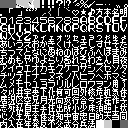News: 11 March 2016 - Forum Rules
Current Moderators - DarkSol, KingMike, MathOnNapkins, Azkadellia, DankeAuthor Topic: Kanji identification  (Read 2267 times)

FCandChill

• Hero Member
•• Posts: 529Kanji identification
« on: September 23, 2018, 02:59:44 pm »
Hi all. I'm making an script editor for a game. Here's the bank with all the characters...And here's the table file I have to far...

Code: [Select]
00=[BLACK 1]
01=[BLACK 2]
02=[???1]
03=[???2]
04=[???3]
05=！
06=[！！]
07=？
08=[！？]
09=[TILE 09]
0A=％
0B=／
0C=―
0D=‥
0E=゛
0F=゜
10=．
11=「
12=」
13=“
14=”
15=[WINDOW 15]
16=[WINDOW 16]
17=[WINDOW 17]
18=[WINDOW 18]
19=[Heart]
1A=★
1B=♪
1C=[KANJI 1C]
1D=[KANJI 1D]
1E=[KANJI 1E]
1F=[KANJI 1F]
20=０
21=１
22=２
23=３
24=４
25=５
26=６
27=７
28=８
29=９
2A=Ａ
2B=Ｂ
2C=Ｃ
2D=Ｄ
2E=Ｅ
2F=Ｆ
30=Ｇ
31=Ｈ
32=Ｉ
33=Ｊ
34=Ｋ
35=Ｌ
36=Ｍ
37=Ｎ
38=Ｏ
39=Ｐ
3A=Ｑ
3B=Ｒ
3C=Ｓ
3D=Ｔ
3E=Ｕ
3F=Ｖ
40=Ｗ
41=Ｘ
42=Ｙ
43=Ｚ
44=ァ
45=ィ
46=ェ
47=ォ
48=ッ
49=ャ
4A=ュ
4B=ョ
4C=っ
4D=ゃ
4E=ゅ
4F=ょ
50=あ
51=い
52=う
53=え
54=お
55=か
56=き
57=く
58=け
59=こ
5A=さ
5B=し
5C=す
5D=せ
5E=そ
5F=た
60=ち
61=つ
62=て
63=と
64=な
65=に
66=ぬ
67=ね
68=の
69=は
6A=ひ
6B=ふ
6C=へ
6D=ほ
6E=ま
6F=み
70=む
71=め
72=も
73=や
74=ゆ
75=よ
76=ら
77=り
78=る
79=れ
7A=ろ
7B=わ
7C=を
7D=ん
7E=[KANJI 7E]
7F=[KANJI 7F]
80=ア
81=イ
82=ウ
83=エ
84=オ
85=カ
86=キ
87=ク
88=ケ
89=コ
8A=サ
8B=シ
8C=ス
8D=セ
8E=ソ
8F=タ
90=チ
91=ツ
92=テ
93=ト
94=ナ
95=ニ
96=ヌ
97=ネ
98=ノ
99=ハ
9A=ヒ
9B=フ
9C=ヘ
9D=ホ
9E=マ
9F=ミ
A0=ム
A1=メ
A2=モ
A3=ヤ
A4=ユ
A5=ヨ
A6=ラ
A7=リ
A8=ル
A9=レ
AA=ロ
AB=ワ
AC=ヲ
AE=[KANJI AE]
AF=[KANJI AF]
B0=々
B1=[KANJI B1]
B2=[KANJI B2]
B3=[KANJI B3]
B4=[KANJI B4]
B5=[KANJI B5]
B6=[KANJI B6]
B7=[KANJI B7]
B8=[KANJI B8]
B9=[KANJI B9]
BA=[KANJI BA]
BB=[KANJI BB]
BC=[KANJI BC]
BD=[KANJI BD]
BE=[KANJI BE]
BF=[KANJI BF]
C0=[KANJI C0]
C1=[KANJI C1]
C2=[KANJI C2]
C3=[KANJI C3]
C4=[KANJI C4]
C5=[KANJI C5]
C6=[KANJI C6]
C7=[KANJI C7]
C8=[KANJI C8]
C9=[KANJI C9]
CA=[KANJI CA]
CB=[KANJI CB]
CC=[KANJI CC]
CD=[KANJI CD]
CE=[KANJI CE]
CF=[KANJI CF]
D0=[KANJI D0]
D1=[KANJI D1]
D2=[KANJI D2]
D3=[KANJI D3]
D4=[KANJI D4]
D5=[KANJI D5]
D6=[KANJI D6]
D7=[KANJI D7]
D8=[KANJI D8]
D9=[KANJI D9]
DA=[KANJI DA]
DB=[KANJI DB]
DC=[KANJI DC]
DD=[KANJI DD]
DE=[KANJI DE]
DF=[KANJI DF]
E0=[KANJI E0]
E1=[KANJI E1]
E2=[KANJI E2]
E3=[KANJI E3]
E4=[KANJI E4]
E5=[KANJI E5]
E6=[KANJI E6]
E7=[KANJI E7]
E8=[KANJI E8]
E9=[KANJI E9]
EA=[KANJI EA]
EB=[KANJI EB]
EC=[KANJI EC]
ED=[KANJI ED]
EE=[KANJI EE]
EF=[KANJI EF]
F0=[KANJI F0]
F1=[KANJI F1]
F2=[KANJI F2]
F3=[KANJI F3]
F4=[KANJI F4]
F5=[KANJI F5]
F6=[KANJI F6]
F7=[KANJI F7]
F8=[KANJI F8]
F9=[KANJI F9]
FA=[KANJI FA]
FB=[KANJI FB]
FC=
FD=
FE=
FF=[BLACK 3]
« Last Edit: September 25, 2018, 06:16:56 pm by FCandChill »

tashi

• Jr. Member
•• Posts: 61Re: Kaji identification
« Reply #1 on: September 23, 2018, 09:40:11 pm »
I'm afraid I'm at a loss for AF=[KANJI AF]
Maybe if you see it in context or in-game or something I might recognize it.

I wonder if a different tile editor would make the characters more readable?
The main block is in alphabetical order so it was pretty easy to guess the characters, but it would've been difficult if they were unsorted.

This is the main block:
々以井宇英下化何可回外核危机気休

And the interspersed ones:
Code: [Select]
1C=[KANJI 1C]
1D=[KANJI 1D]
1E=[KANJI 1E]
1F=[KANJI 1F]
...
7E=[KANJI 7E]
7F=[KANJI 7F]
...
AE=[KANJI AE]

FCandChill

• Hero Member
•• Posts: 529Re: Kanji identification
« Reply #2 on: September 25, 2018, 07:36:22 am »
I'm afraid I'm at a loss for AF=[KANJI AF]
Maybe if you see it in context or in-game or something I might recognize it.

Thanks for the help! Here's one of the lines that uses the [KANJI AF]...

Code: [Select]
―　メタルスレイタ゛―　全き発かんしゅうふつう[KANJI AF]　―
« Last Edit: September 25, 2018, 06:17:05 pm by FCandChill »

tashi

• Jr. Member
•• Posts: 61Re: Kanji identification
« Reply #3 on: September 25, 2018, 07:31:49 pm »
It's probably 了 ... doesn't exactly make a lot of sense that I can tell. But it's probably right.

FCandChill

• Hero Member
•• Posts: 529Re: Kanji identification
« Reply #4 on: September 25, 2018, 07:41:44 pm »
Thanks, but it seems like you're unsure. Here's all the lines that use this character...

Code: [Select]
―　メタルスレイタ゛―　全き発かんしゅうふつう[KANJI AF]　―また　　―　ふたい　てきと　せっしょくらないしますひとつ　―また
Code: [Select]
[KANJI AF]かい！‥‥忠さん

し゛かんをくいます

てきか゛きたらわ　こちらから

うってか゛　入っ出ましょう゛か

Code: [Select]
とウ゛ィウ゛ァ―チェは[KANJI AF]かい！と‥‥と何か゛　はし゛まるんた゛ろ‥‥やっ[TILE 09]し゛ゃ　ないて゛

た゛め　　きかない‥‥

とつきゃっ　くっ　くる！！

Code: [Select]
‥‥[KANJI AF]かい‥‥
Code: [Select]
[KANJI AF]かい！
Code: [Select]
[KANJI AF]かい！

‥‥

て゛も‥‥あっ　き　きます！

tashi

• Jr. Member
•• Posts: 61Re: Kanji identification
« Reply #5 on: September 25, 2018, 10:32:19 pm »
Yeah, it's 100% 了
The font made it kinda hard to tell. I would've expected it to look like the 子 a few lines below it, just without the middle horizontal line, but the tiny font tile makes it look completely different, shifted to the right and shorter on top.

The tone of the first example line you gave made me think military-ish, so they'd probably say 終了 and 了解 and stuff, but I don't understand what they're actually saying, so I couldn't be sure from that alone.

Every additional sample you shared is 了解　了かい or "roger" so I'm confident it's correct now.

FCandChill

• Hero Member
•• Posts: 529Re: Kanji identification
« Reply #6 on: September 26, 2018, 09:42:41 pm »
Thanks for the help, tashi!